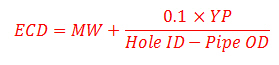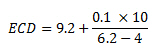# Equivalent Circulating Density (ECD) Using Yield Point for MW less than 13 ppg

Another way to determine equivalent circulating density (ECD) is to use yield point.This formula below is used to calculate the ECD and it is good for mud weight less than or equal to 13.0 ppg.Where:

ECD is equivalent circulating density in ppg

MW is mud weight in ppg

Hold ID is inside diameter of hole in inch

Pipe OD is outside diameter of pipe in inch

YP is mud yield point

YP can be calculated by these following equations

YP = Reading at 300 rpm – PV

PV = Reading at 600 rpm – Reading at 300 rpm

Determine the ECD with the following information

MW = 9.2 ppg

Reading at 300 = 25

Reading at 600 = 40

Hole diameter = 6.2

Pipe diameter = 4

PV = 40 – 25 = 15

YP = 25- 15 = 10ECD = 9.7 ppg

Additional articles about Equivalent Circulating Density (ECD) are as follows:

Equivalent Circulating Density (ECD) in ppg

Equivalent Circulation Density (ECD) with complex engineering equations

Effect of Frictional Pressure on ECD while reverse circulation

Effect of Frictional Pressure on ECD while forward circulation

Share the joyWorking in the oil field and loving to share knowledge.

### 4 Responses to Equivalent Circulating Density (ECD) Using Yield Point for MW less than 13 ppg

1.Theophilus D.B. Duudee says:

Too good a lesson for me. This is my field of study. Each time i comes across Drilling formulers.com, i feel good. Their teaching inspairs me, couple with my Drilling and Well service technology study in GODAC Ghana. U ar hundred percent ok. More grees to ur elbows.

2.roberto ruiz gonzalez says:

este tema me ha sido de gran ayuda , afortunadamente en los campos geotermicos no tenemos problemas con los reventones , asi que es poco usual coo en la industria del petroleo. saludos todos desde mexicali,baja california ,mexico

3.Ahmed Sariakousy says:

Good , but as I see this is calculated estimating that you have only one section of pipe and hole only . what about the BHA section , and what about if we have 2 types of pipes like 5.5 inch and 4 inch ….ect ? how can I apply this formula ?

•DrillingFormulas.Com says:

Ahmed,

For this case, I will use 5.5 inch because it will give you the worst case calculation.

Best Regards,
Shyne.

This site uses Akismet to reduce spam. Learn how your comment data is processed.